HomeForum of Mathematics, Sigma

# SECOND MOMENTS IN THE GENERALIZED GAUSS CIRCLE PROBLEM

Published online by Cambridge University Press:  19 December 2018

Corresponding

## Abstract

The generalized Gauss circle problem concerns the lattice point discrepancy of large spheres. We study the Dirichlet series associated to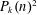$P_{k}(n)^{2}$ , where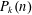$P_{k}(n)$ is the discrepancy between the volume of the$k$ -dimensional sphere of radius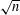$\sqrt{n}$ and the number of integer lattice points contained in that sphere. We prove asymptotics with improved power-saving error terms for smoothed sums, including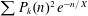$\sum P_{k}(n)^{2}e^{-n/X}$ and the Laplace transform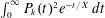$\int _{0}^{\infty }P_{k}(t)^{2}e^{-t/X}\,dt$ , in dimensions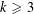$k\geqslant 3$ . We also obtain main terms and power-saving error terms for the sharp sums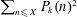$\sum _{n\leqslant X}P_{k}(n)^{2}$ , along with similar results for the sharp integral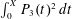$\int _{0}^{X}P_{3}(t)^{2}\,dt$ . This includes producing the first power-saving error term in mean square for the dimension-3 Gauss circle problem.

## MSC classification

Type
Research Article
Information
Creative Commons
This is an Open Access article, distributed under the terms of the Creative Commons Attribution licence (http://creativecommons.org/licenses/by/4.0/), which permits unrestricted re-use, distribution, and reproduction in any medium, provided the original work is properly cited.

## References

Bourgain, J. and Watt, N., ‘Mean square of zeta function, circle problem and divisor problem revisited’. arXiv preprint, arXiv:1709.04340 (2017).Google Scholar
Choi, S. K. K., Kumchev, A. V. and Osburn, R., ‘On sums of three squares’, Int. J. Number Theory 1(2) (2005), 161173.CrossRefGoogle Scholar
Deshouillers, J.-M. and Iwaniec, H., ‘Kloosterman sums and Fourier coefficients of cusp forms’, Invent. Math. 70(2) (1982/83), 219288.CrossRefGoogle Scholar
Goldfeld, D., Automorphic Forms and L-functions for the Group GL(n, R), Cambridge Studies in Advanced Mathematics, 99 (Cambridge University Press, Cambridge, 2015), With an appendix by Kevin A. Broughan, Paperback edition of the 2006 original.Google Scholar
Gradshteyn, I. S. and Ryzhik, I. M., Table of Integrals, Series, and Products, 8th edn (Elsevier/Academic Press, Amsterdam, 2015), Translated from the Russian, Translation edited and with a preface by Daniel Zwillinger and Victor Moll, Revised from the seventh edition.Google Scholar
Gupta, S. D., ‘Corrigendum to my paper ‘The Rankin–Selberg method on congruence subgroups’’, Illinois J. Math. 44(4) (2000), 924926.Google Scholar
Gupta, S. D., ‘The Rankin–Selberg method on congruence subgroups’, Illinois J. Math. 44(1) (2000), 95103.Google Scholar
Heath-Brown, D. R., ‘Lattice points in the sphere’, inNumber Theory in Progress, Vol. 2 (Zakopane-Kościelisko 1997) (de Gruyter, Berlin, 1999), 883892.Google Scholar
Hoffstein, J. and Hulse, T. A., ‘Multiple Dirichlet series and shifted convolutions’, J. Number Theory 161 (2016), 457533. With an appendix by Andre Reznikov.CrossRefGoogle Scholar
Hulse, T. A., Kuan, C. I., Lowry-Duda, D. and Walker, A., ‘The Laplace transform of the second moment in the Gauss circle problem’. arXiv preprint, arXiv:1705.04771 (2017).Google Scholar
Hulse, T. A., Kuan, C. I., Lowry-Duda, D. and Walker, A., ‘The second moment of sums of coefficients of cusp forms’, J. Number Theory 173 (2017), 304331.CrossRefGoogle Scholar
Hulse, T. A., Kuan, C. I., Lowry-Duda, D. and Walker, A., ‘Short-interval averages of sums of Fourier coefficients of cusp forms’, J. Number Theory 173 (2017), 394415.CrossRefGoogle Scholar
Huxley, M. N., ‘Introduction to Kloostermania’, inElementary and Analytic Theory of Numbers (Warsaw, 1982), Banach Center Publ., 17 (PWN, Warsaw, 1985), 217306.Google Scholar
Huxley, M. N., ‘Exponential sums and lattice points. III’, Proc. Lond. Math. Soc. (3) 87(3) (2003), 591609.CrossRefGoogle Scholar
Ivić, A., ‘A note on the Laplace transform of the square in the circle problem’, Studia Sci. Math. Hungar. 37(3–4) (2001), 391399.Google Scholar
Ivić, A., Krätzel, E., Kühleitner, M. and Nowak, W. G., ‘Lattice points in large regions and related arithmetic functions: recent developments in a very classic topic’, inElementare und analytische Zahlentheorie, Publications of the Scientific Society at the Johann Wolfgang Goethe University, Frankfurt am Main. Franz Steiner Verlag Stuttgart, Postfach 10 10 61, 70009 Stuttgard, Germany, 20 (Franz Steiner Verlag Stuttgart, Stuttgart, 2006), 89128.Google Scholar
Iwaniec, H., Spectral Methods of Automorphic Forms, Graduate Studies in Mathematics, 53 (American Mathematical Society, Providence, RI, 2002), Revista Matemática Iberoamericana, Madrid, second edition.CrossRefGoogle Scholar
Iwaniec, H. and Kowalski, E., Analytic Number Theory, American Mathematical Society Colloquium Publications, 53 (American Mathematical Society, Providence, RI, 2004).Google Scholar
Jarník, V., ‘Über die Mittelwertsätze der Gitterpunktlehre. V’, Časopis Pěst. Mat. Fys. 69 (1940), 148174.Google Scholar
Kıral, E. M., ‘Subconvexity for half integral weight L-functions’, Math. Z. 281(3–4) (2015), 689722.CrossRefGoogle Scholar
Krätzel, E., Analytische Funktionen in der Zahlentheorie, Teubner-Texte zur Mathematik [Teubner Texts in Mathematics], 139 (B. G. Teubner, Stuttgart, 2000).CrossRefGoogle Scholar
Kubota, T., Elementary Theory of Eisenstein Series (Kodansha Ltd., Tokyo; Halsted Press [John Wiley & Sons], New York–London–Sydney, 1973).Google Scholar
Landau, E., ‘Über die Gitterpunkte in einem Kreise’, Math. Z. 5(3–4) (1919), 319320.CrossRefGoogle Scholar
Landau, E., Vorlesungen über Zahlentheorie. zweiter Band (Chelsea Publishing Co., New York, 1969).Google Scholar
Lau, Y.-K., ‘On the mean square formula of the error term for a class of arithmetical functions’, Monatsh. Math. 128(2) (1999), 111129.CrossRefGoogle Scholar
Lau, Y.-K. and Tsang, K.-M., ‘On the mean square formula of the error term in the Dirichlet divisor problem’, Math. Proc. Cambridge Philos. Soc. 146(2) (2009), 277287.CrossRefGoogle Scholar
Montgomery, H. L. and Vaughan, R. C., Multiplicative Number Theory I: Classical Theory, Vol. 97 (Cambridge University Press, Cambridge, 2006).CrossRefGoogle Scholar
Müller, W., ‘The mean square of Dirichlet series associated with automorphic forms’, Monatsh. Math. 113(2) (1992), 121159.CrossRefGoogle Scholar
Sierpiński, W., ‘O pewnem zagadnieniu z rachunku funckcyi asymptotycnych’, Prace mat.-fiz (1906), 77118.Google Scholar
Vinogradov, I. M., ‘On the number of integer points in a sphere’, Izv. Akad. Nauk SSSR Ser. Mat. 27 (1963), 957968.Google Scholar
Zagier, D., ‘The Rankin–Selberg method for automorphic functions which are not of rapid decay’, J. Fac. Sci. Univ. Tokyo Sect. IA Math. 28(3) (1982), 415437. 1981.Google Scholar

### Full text views

Full text views reflects PDF downloads, PDFs sent to Google Drive, Dropbox and Kindle and HTML full text views.

Total number of HTML views: 0
Total number of PDF views: 222 *
View data table for this chart

* Views captured on Cambridge Core between 19th December 2018 - 25th January 2021. This data will be updated every 24 hours.

Access
Open access
Hostname: page-component-898fc554b-gwqw7 Total loading time: 0.459 Render date: 2021-01-25T11:19:06.831Z Query parameters: { "hasAccess": "1", "openAccess": "1", "isLogged": "0", "lang": "en" } Feature Flags: { "shouldUseShareProductTool": true, "shouldUseHypothesis": true, "isUnsiloEnabled": true, "metricsAbstractViews": false, "figures": false, "newCiteModal": false }

# Send article to Kindle

Note you can select to send to either the @free.kindle.com or @kindle.com variations. ‘@free.kindle.com’ emails are free but can only be sent to your device when it is connected to wi-fi. ‘@kindle.com’ emails can be delivered even when you are not connected to wi-fi, but note that service fees apply.

Find out more about the Kindle Personal Document Service.

SECOND MOMENTS IN THE GENERALIZED GAUSS CIRCLE PROBLEM
Available formats
×

# Send article to Dropbox

To send this article to your Dropbox account, please select one or more formats and confirm that you agree to abide by our usage policies. If this is the first time you use this feature, you will be asked to authorise Cambridge Core to connect with your <service> account. Find out more about sending content to Dropbox.

SECOND MOMENTS IN THE GENERALIZED GAUSS CIRCLE PROBLEM
Available formats
×

# Send article to Google Drive

To send this article to your Google Drive account, please select one or more formats and confirm that you agree to abide by our usage policies. If this is the first time you use this feature, you will be asked to authorise Cambridge Core to connect with your <service> account. Find out more about sending content to Google Drive.

SECOND MOMENTS IN THE GENERALIZED GAUSS CIRCLE PROBLEM
Available formats
×
×